## Transfer Function

The engineering terminology for one use of Fourier Transforms. By breaking up a wave pulse into its frequency spectrum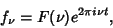(1)

the entire signal can be written as a sum of contributions from each frequency,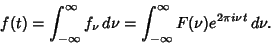(2)

If the signal is modified in some way, it will become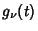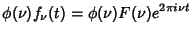(3)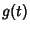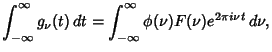(4)

where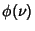is known as the transfer function.'' Fourier Transforming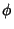and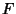,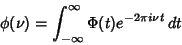(5)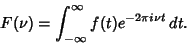(6)

From the Convolution Theorem,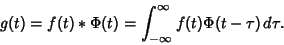(7)